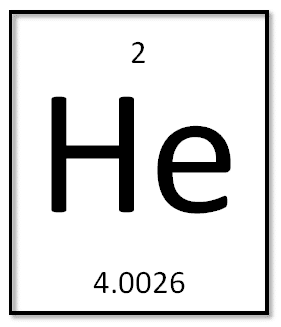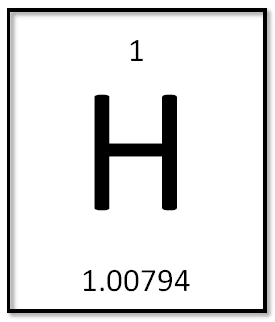SearchSearch

# Understanding units of measure for specialty gas mixturesThe compositions of gas mixtures are expressed in a number of different ways. These can change depending upon the mixture’s method of manufacture, the type of components within the mixture, the amount of each component, the process/analytical requirements, or simply the customer’s preference.

In each case the units of measure and the ratio of these units relative to each other are listed on the certificate provided by the manufacturer.

• Gas mixtures are commonly certified using the following units of measure:
• mol Moles
• vol Volume
• mg/g/kg Milligrams/grams/kilograms

These units are then given relative values using percentage ratios, which can be listed in a number of different ways. You may see one of these commonly used ratios:

• ppb Parts per billion
• ppm Part per million
• % Percentage

On their own, these ratios are meaningless. We must combine them with a unit of measure to derive their significance.  Some commonly used ways to display units & ratios together:

• mg/kg Milligrams per kilogram
• wt/wt Weight per total weight
• ppmv Parts per million, volume
• %vol, or %v/v Percentage of volume, percent volume per volume.
• %mol Percentage of moles

It is important to understand the reasons for using each of the various methods of measurement and to understand the difference between the different units of measure so we can be sure to recognise the most appropriate type for any particular application.

Moles are commonly used when preparing gas mixtures gravimetrically. A mole is a unit of measure used to describe an amount of a chemical, based on its molecular weight, or molar mass. The molar mass is the weight of a single particle in its most elementary form (e.g. atoms or molecules). The atomic weight of each element is different, and is expressed as the number of grams per mole. You can see this number displayed on the period table of elements. Take the second element, helium, as an example:Helium’s atomic weight is 4.0026 grams per mole. Helium’s molar mass is also 4.0026 grams per mole, since its most elementary form is a single atom. However, some elements do not exist as a single atom, and so the molar mass is different to the atomic weight. This is the case with Hydrogen, the first element:Hydrogen’s atomic weight is 1.00794 grams per mole. But since hydrogen exists as H2 (H + H), we double the atomic weight to get the molar mass of 2.01588 grams per mole.

This is important for gravimetrically prepared gas mixtures, because all calculations are done based on the weight of each component added. When mixtures are described with moles, the total moles of all components are added together, and then each component is listed as a ratio of the total moles in the mixture.

Milligrams, grams, and kilograms can also be used when preparing mixture gravimetrically. However, instead of calculating the number of moles, the actual weight of each component is used to create the ratios.

Volume, as a unit, is most typically used in volumetrically prepared gas mixtures, though it can be used elsewhere. Each gas in a mixture will occupy a certain amount of space at a certain pressure and temperature, and the amount of space occupied by each gas is described using volume as a unit. This is inherently less accurate than using moles or grams, because each gas will expand and contract relative to pressure and temperature, and at different rates, whereas moles and grams will always remain constant. This means that when using volume, and depending on the environment in which you’re using the mixture, the mixture ratios can change. For this reason, manufacturers will normally include a set of reference conditions: temperature and pressure, sometimes expressed as “STP” – Standard Temperature and Pressure. A volumetric certification is only accurate at the exact temperature and pressure specified on the certificate. If used outside of these conditions, the relative composition will change.

Sometimes, hybrid measurements are used. For example, milligrams per cubic metre (mg/m3), which is a mass measurement within a volumetric measurement. In such cases, the user must be aware that the same potential inaccuracies which apply to purely volumetric measurements are inherent in such a hybrid measurement, and adhering to the reference conditions is vital for accurate calibration.

Conversions between volumetric and gravimetric measurements can be made, but only when a set of reference conditions are included. It is possible to produce a mixture gravimetrically, but provide certification based on a volumetric unit. But, this certification would only be accurate if the gas was used at the exact set of reference conditions specified.

## Request a quote

Request a quote today for our speciality gas products for your specific needs. Enjoy high quality products, competitive products & fast delivery.

Scroll to Top